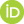# All science as rigorous science: the principle of constructive mathematizability of any theory

Penchev, Vasil (2020) All science as rigorous science: the principle of constructive mathematizability of any theory. [Preprint]Preview
Text
@SaltLakeSityUltimateNamed.pdf

## Abstract

A principle, according to which any scientific theory can be mathematized, is investigated. Social science, liberal arts, history, and philosophy are meant first of all. That kind of theory is presupposed to be a consistent text, which can be exhaustedly represented by a certain mathematical structure constructively. In thus used, the term “theory” includes all hypotheses as yet unconfirmed as already rejected. The investigation of the sketch of a possible proof of the principle demonstrates that it should be accepted rather a metamathematical axiom about the relation of mathematics and reality.
The main statement is formulated as follows: Any scientific theory admits isomorphism to some mathematical structure in a way constructive (that is not as a proof of “pure existence” in a mathematical sense).
Its investigation needs philosophical means. Husserl’s phenomenology is what is used, and then the conception of “bracketing reality” is modelled to generalize Peano arithmetic in its relation to set theory in the foundation of mathematics. The obtained model is equivalent to the generalization of Peano arithmetic by means of replacing the axiom of induction with that of transfinite induction.
The sketch of the proof is organized in five steps: (1) a generalization of epoché; (2) involving transfinite induction in the transition between Peano arithmetic and set theory; (3) discussing the finiteness of Peano arithmetic; (4) applying transfinite induction to Peano arithmetic; (5) discussing an arithmetical model of reality.
Accepting or rejecting the principle, two kinds of mathematics appear differing from each other by its relation to reality. Accepting the principle, mathematics has to include reality within itself in a kind of Pythagoreanism. These two kinds are called in paper correspondingly Hilbert mathematics and Gödel mathematics. The sketch of the proof of the principle demonstrates that the generalization of Peano arithmetic as above can be interpreted as a model of Hilbert mathematics into Gödel mathematics therefore showing that the former is not less consistent than the latter, and the principle is an independent axiom.
The present paper follows a pathway grounded on Husserl’s phenomenology and “bracketing reality” to achieve the generalized arithmetic necessary for the principle to be founded in alternative ontology, in which there is no reality external to mathematics: reality is included within mathematics. That latter mathematics is able to self-found itself and can be called Hilbert mathematics in honour of Hilbert’s program for self-founding mathematics on the base of arithmetic.
The principle of universal mathematizability is consistent to Hilbert mathematics, but not to Gödel mathematics. Consequently, its validity or rejection would resolve the problem which mathematics refers to our being; and vice versa: the choice between them for different reasons would confirm or refuse the principle as to the being.
An information interpretation of Hilbert mathematics is involved. It is a kind of ontology of information. The Schrödinger equation in quantum mechanics is involved to illustrate that ontology. Thus the problem which of the two mathematics is more relevant to our being (rather than reality for reality is external only to Gödel mathematics) is discussed again in a new way
A few directions for future work can be: a rigorous formal proof of the principle as an independent axiom; the further development of information ontology consistent to both kinds of mathematics, but much more natural for Hilbert mathematics; the development of the information interpretation of quantum mechanics as a mathematical one for information ontology and thus Hilbert mathematics; the description of consciousness in terms of information ontology.

 Export/Citation: EndNote | BibTeX | Dublin Core | ASCII/Text Citation (Chicago) | HTML Citation | OpenURL
 Social Networking:

Item Type: Preprint
Creators:
CreatorsEmailORCIDPenchev, Vasilvasildinev@gmail.com
Keywords: axiom of choice; axiom of induction; axiom of transfinite induction; eidetic, phenomenological and transcendental reduction; epoché; Gödel mathematics; Hilbert mathematics; information; quantum mechanics, quantum information; phenomenology; principle of universal mathematizability
Subjects: Specific Sciences > Mathematics > Logic
General Issues > Scientific Metaphysics
General Issues > Structure of Theories
Depositing User: Prof. Vasil Penchev
Date Deposited: 22 Apr 2020 14:56
Item ID: 17102
Subjects: Specific Sciences > Mathematics > Logic
General Issues > Scientific Metaphysics
General Issues > Structure of Theories
Date: 22 April 2020
URI: http://philsci-archive.pitt.edu/id/eprint/17102

### Monthly Views for the past 3 yearsView Item